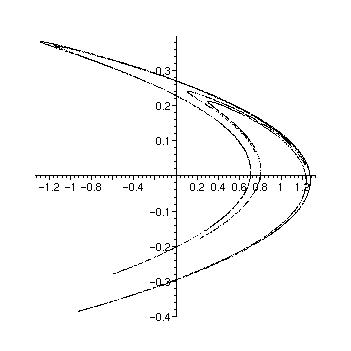Advice: Plotting a sequence of points

Suppose you want to produce a plot by generating points one at a time, and plotting them. In a typical programming language such as C, you would probably build up the plot one point at a time, plotting each point as it is generated. This is not the way Maple works, however. In Maple a plot is a single object, whose image on the screen or in a file is "printed" as the result of one command (although this may use the results of several different plot commands, combined with display ).

To plot a sequence of points, you build up a list or array of points and then plot it. In the case of a large number of points, an array can be more efficient. It is also faster to use pointplot in the plots package rather than plot with the style=POINT option. This procedure can handle an array directly, without converting it to a list of lists (which would be required by plot ). Similarly, for a three-dimensional plot you can use pointplot3d in the plots package.

Use the option
symbol=POINT when plotting points to make each point be a single dot. The other possibilities are BOX , CIRCLE , CROSS (which is the default) and DIAMOND . 

Example:

Here we generate a plot of 4000 successive points of the Hénon map . To take maximum advantage of the speed of hardware floating-point arithmetic, we write a procedure to compute the points and put them in an array, and evaluate it using evalhf .

> makearray:= proc(L)
local i, x, y, xp;
x:= 0.6; y := 0.2;
for i to 400 do
xp := 1+y-1.4*x^2;
y := .3*x;
x := xp;
od;
for i to 4000 do
xp := 1+y-1.4*x^2;
y := .3*x;
x := xp;
L[i,1]:= x; L[i,2]:= y;
od;
end:

We construct the array, and pass it "by reference" to the procedure with the var construction so that the procedure can modify it.

> L:= array(1..4000,1..2):
evalhf(makearray(var(L))):

Now we use pointplot to plot the array of points.

> plots[pointplot](L,symbol=POINT);